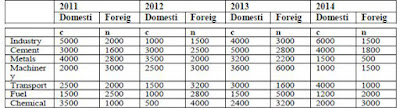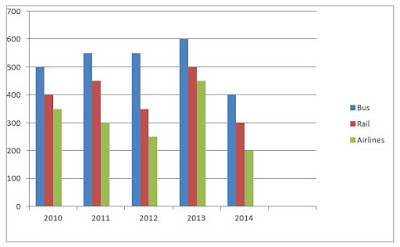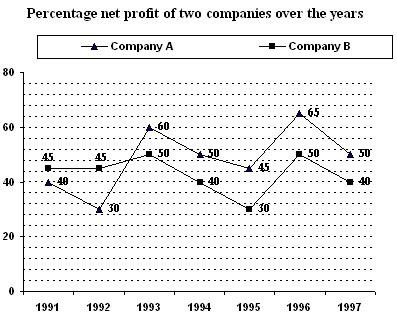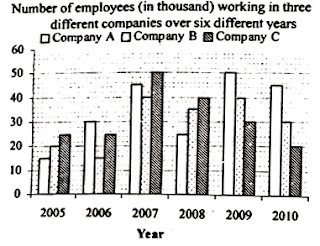Showing posts with label Quantitative Aptitude. Show all posts
Showing posts with label Quantitative Aptitude. Show all posts

### Data Interpretation Quiz

Direction(01-05): Study the given table carefully to answer the following questions:

Following table shows the investment (In Rs. Crore) in various sectors in different years### Quant Quiz- Data Interpretation

Directions (Q. 1 - 5): Read the table carefully to answer the questions that follows:

Percentage wise share distribution is given of different companies P, Q, R, S and T out of the total shares### Data Interpretation Questions with Solution

Directions (1 to 5): Study the following information carefully and answer the questions given below:

## Data analysis and Interpretation

Directions (Q.1-5): Study the given table carefully to answer the given questions.
Percentage profit or loss is based on the sum of cost price transportation cost.### Quant Quiz- Simplification and Quadratic Equation for IBPS and Other Exams

Dear Bank Aspirants practice Quant Questions for upcoming SBI and other exams. Today's Topic is  Simplification and Quadratic Equation, very important for IBPS clerk point of view.

### Quantitative Aptitude Questions for IBPS RRB PO and Clerk Exam 2017

Directions (1-10): In the following number series only one number is wrong. Find out the wrong number.

1. 9050, 5675, 3478, 2147, 1418, 1077, 950
(a) 3478
(b) 1418
(c) 5675
(d) 2147
(e) 1077

### Data Interpretation for Bank Exams

Directions (Q.1-5): Study the following bar graph carefully and answer the questions given below:

The graph shows the no. of persons using different modes of transport over the years.## New Pattern Questions of Quantitative For IBPS RRB

### Data Interpretation for IBPS Exams 2017

Direction (01-05): Study the following graph carefully and answer the questions given below:### Quant Quiz for SBI PO

Directions (1-5): What value should come in the place of question mark (?) in the following questions?### Quant Quiz for SBI PO, IBPS Exams

Directions (1-6): Refer to the pie-charts and answer the given questions:

### Data Interpretation for Clerk Mains, IPPB

Directions (01-05) : Study the following pie chart and table and answer accordingly.

The following pie-charts show the percentage of employees in two companies X and Y who are working in different units. Total numbers of employees in X and Y are 2500 and 3000 respectively.The following table shows the ratio of males and females of both the companies in different units1). In which of the following units, the number of male employees of company X is equal to that of female employees of company Y ?
a) C
b) F
c) A
d) E
e) B

2). By what per cent is the number of female employees of company Y for unit E more than that of male employees of company X for same unit ?
a) 34%
b) 50%
c) 46.66%
d) 54.54%
e) None of these

3). In which of the following two units, the number of male employees for company Y is the same ?
a) A and B
b) A and C
c) D and E
d) B and C
e) None of these

4). Find the ratio of the male employees in unit E of company X to the female employees in unit C of company Y.
a) 2 : 5
b) 3 : 2
c) 2 : 3
d) 1 : 3
e) None of these

5). In how many units of company Y are the female employees more than 200 ?
a) Four
b) Five
c) Six
d) Three
e) None of these

Directions (06–10) :
The following line-graph shows the number of males and females of two different towns X and Y during the given years:

6). By what percent (in approx) the average population of both the towns has increased during the period 1994 to 1998 ?
a) 5%
b) 8%
c) 7%
d) 4%
e) None of these

7). Find the ratio between the average number of males of X and Y for the given period.
a) 237 : 230
b) 239 : 230
c) 49 : 48
d) 230 : 237
e) None of these

8). The population of X and Y in 1996 is how many times of 1994 ?
a) 1.05
b) 0.94
c) 1.02
d) 0.91
e) None of these

9). Find the number of years in which the number of females for X and Y are less than their respective average numbers.
a) 0
b) 4
c) 3
d) 5
e) None of these

10). In which of the following year the difference between the number of females in town X and number of males in town Y is maximum?
a) 1994
b) 1995
c) 1998
d) 1996
e) 1997

1. (A); Number of male employees in unit C for company X
= 14% of 2500 × (3/7) = 150
Number of female employees in unit C for company Y
= 15% of 3000 × (1/3) = 150

2. (E); Number of female employees of company Y in unit E
= 22% of 3000 × 1/3 = 220
Number of male employees of company X in unit E
= 10% of 2500 × 2/5 = 100
:. Required more % = (220-100)/100 × 100
= 120%

3. (B); Number of male employees in different units for company Y : A = 300,
B = 240, C = 300, D = 100, E = 440 and F = 150.
Hence, our answer is A and C.

4. (C); Required ratio = (10%of 2500×2/5) / (15% of 3000×1/3)= 2 : 3

5. (A); Number of female employees for company Y in different units:
A = 60, B = 300, C = 150, D = 500, E = 220 and F = 240

6. (C); Average population (in thousands) of both the towns in 1994
= (45+44+48+45)/2
= 45 + 22 + 24 = 91 and that in 1998
= (50+47+50+47)/ 2
= 50 + 47 = 97
:. Required percentage increase = (6/91) × 100
= 6.59% Approx. 7%.

7. (B); Required ratio

8. (A); Population of X and Y in 1996
= (50 + 47+47 + 48 ) × 1000 = 192000
Population of X and Y in 1994
= (45 + 44 + 48 + 45) × 1000 = 182000
Now, 192 × 1000 = x × 182 × 1000
:. x = (192/182) = 1.05

9. (B); Average number of females for
X =1/5 (45 + 47 + 48 + 50 + 47) × 1000
= 237 × 200 = 47400
Y =1/5 (48 + 49 + 47 + 50 + 50) × 1000
= 244 × 200 = 48800
So, 1994, 1995 and 1998 are the three desired years for X and 1994 and 1996
are the two desired years for Y. Hence the required number of years is four
(1994, 1995, 1996 and 1998).

10. (B)
In 1994 → (45000 – 44000) = 1000
In 1995 → (47000 - 44000) = 3000
In 1996 → (48000 – 47000) = 1000
In 1997 → (50000 – 48000) = 2000
In 1998 → (47000 – 47000) = 0

### Quant Quiz for IBPS Clerk Mains, India Post

Directions (Q. 1 - 5): In each of the following questions, a series of numbers which contains a wrong number is given. Find out the wrong number in each case.

### India Post, IBPS Clerk-Data Interpretation Quiz

Directions (Q. 01-05): Study the following graph carefully and answer the questions given below:1). The total number of employees in Company A over the years together was approximately what per cent of the total number of employees in Company C in the year 2007 and 2008 together?
a) 210%
b) 225%
c) 45%
d) 233%
e) 155%

2). What was the percentage decrease in the number of employees in Company B in the year 2008 in comparison to the previous year?
a) 22%
b) 10.5%
c) 12.5%
d) 25%
e) 27.5%

3). What was the ratio of the number of employees in Company B in the year 2005 to the number of the employees in Company C in the year 2007?
a) 5 : 4
b) 2:5
c) 4:5
d) 2:7
e) 3:5

4). If 30 per cent of the total number of employees in the year 2006 in all the companies together were females, what was the total number of male employees that year in all the companies together?
a) 4900
b) 59000
c) 48000
d) 49000
e) 48500

5). What is the ratio of the total employees in all three companies in 2007 to that in 2009?
a) 9 : 8
b) 7:6
c) 5:4
d) 6:5
e) 4:3

Directions Q 6- 10: The bar graph provided below gives the data of the production of paper (in lakh tonnes) by three different companies X, Y, Z over the years. Study the bar chart and answer the questions.6). What is the difference between the production of Company A in 2004 and Company B in 2003?
a) 10,00,000 tonnes
b) 15,00,000 tonnes
c) 20,00,000 tonnes
d) 25,00,000 tonnes
e) 5,00,000 tonnes

7). What is the ratio of the average production of Company C in the period 2002 - 2005 to the average production of company A in the same period?

a) 47:46
b) 49:47
c) 46:47
d) 49:46
e) 46:49

8). What is the percentage increase in the production of Company C from 2003 to 2005?
a) 27%
b) 26%
c) 28%
d) 25%
e) 30%

9). The average production for five years was maximum for which Company?
a) C
b) A
c) B
d) A and C
e) B and C

10). In which year the percentage of production of Company B to the production of company A is maximum?
a) 2004
b) 2002
c) 2001
d) 2005
e) 2003

1. D); Total number of employees in Company A over all the years together
=(15+30+45+25+50+45)x 1000 =210000
Total number of employees in Company C in the year 2007 and 2008
= (50 +40) x 1000=90000
Reqd % = (210000x100/90000)=233.3% =Approx. 233%

2. C); Number of employees in Company B in the year 2008=35000
Number of employees in Company B in the year 2007=40000
%decrease = (40 -35)/40 x (1000/1000) x 100=12.5%

3. B); Number of employees in Company B in the year 2005=20000
Number of employees in Company C in the year 2007=50000
:. Reqd ratio =20000 : 50000 =2 : 5

4. D); Total number of employees in 2006 in all the companies together
=(30 +15 +25)x1000=70000
Number of female employees = 70000 x (30/100) = 21000
:. Number of male employees = (70000 - 21000)
=49000

5. A); Total number of employees in Company A, B and C in 2007
=(45 + 40 + 50) x 1000 =135000
Total number of employees in Company A, B and C in 2009 = (50 + 40 + 30) x 1000
=120000
Reqd ratio = 135000 :120000 =9 : 8

6. b
As per bar chart, Difference will be
[80-65]*100000 = 15,00,000 tonnes
Please note: we multiplied it by 100000, because graph is given in "lakh" tones

7. d
Average production of Company A in the period 2002 – 2005 = ( 55+ 60+ 65+ 50)/4= 57.5
Average production of Company C in the period 2002 – 2005 = (45+ 55+ 75+ 70)/4 =61.25
Required ratio = 61.25 : 57.5 = 2.45 : 2.30 = 49: 46

8. a
Percentage increase in the production of Company C from 2003 to 2005 = ((70-55) / 55) * 100
=(15/55) *100 = 27.27 % ≈ 27%

9. c
Average production of Company A in the period 2001 – 2005 = (70+ 55+ 60+ 65+ 50)/5= 60
Average production of Company B in the period 2001 – 2005 = (55+ 65+ 80+45+ 65)/5= 62
Average production of Company C in the period 2001 – 2005 = (60+45+ 55+ 75+ 70)/5 =61
So company B has the maximum average.

10. e
2001 → % = (55 /70)*100= 78.5 %
2002 → % = (65 / 55)*100= 118.2 %
2003→ % = (80/60)*100= 133.3%
2004→ % = (45/65)*100 = 69.2%
2005→ % = (65/50)*100= 130%
So for 2003 the percentage is highest.

### Quant Quiz for IBPS Clerk Mains, NIACL, India Post

Practice Quant Quiz for IBPS Clerk Mains, NIACL, India Post.

### Quant Quiz-Boat and Stream

Practice Quant Quiz-Boat and Stream for IBPS Clerk Mains, NIACL, India Post.

### Profit and Loss

1.A shopkeeper sells two watches for Rs 308 each. On one watch he earns 12% profit and on the others he suffers 12% loss. His profit or loss in the entire transaction was:
1. 1(11/25)% loss
2. 1(11/25)% gain
3. 3(2/25)% loss
4. 3(2/25)% gain

### Quant Quiz for RRB Mains

Directions (Q. 1-5): What value should come in place of question mark (?) in the following number series?

### Simplification for IBPS Clerk, India Post

Directions (Q. 1–5): What value should come in the place of question mark (?) in the following questions?